This is “Solving Linear Equations: Part II”, section 2.4 from the book Beginning Algebra (v. 1.0). For details on it (including licensing), click here.

Has this book helped you? Consider passing it on:
Creative Commons supports free culture from music to education. Their licenses helped make this book available to you.
DonorsChoose.org helps people like you help teachers fund their classroom projects, from art supplies to books to calculators.

## 2.4 Solving Linear Equations: Part II

### Learning Objectives

1. Solve general linear equations.
2. Identify and solve conditional equations, identities, and contradictions.
3. Clear decimals and fractions from equations.
4. Solve literal equations or formulas for a given variable.

## Combining Like Terms and Simplifying

Linear equations typically are not given in standard form, so solving them requires additional steps. These additional steps include simplifying expressions on each side of the equal sign using the order of operations.

## Same-Side Like Terms

We will often encounter linear equations where the expressions on each side of the equal sign can be simplified. Typically, this involves combining same-side like termsLike terms of an equation on the same side of the equal sign.. If this is the case, then it is best to simplify each side first before solving.

Example 1: Solve: $−4a+2−a=3−2$.

Solution: First, combine the like terms on each side of the equal sign.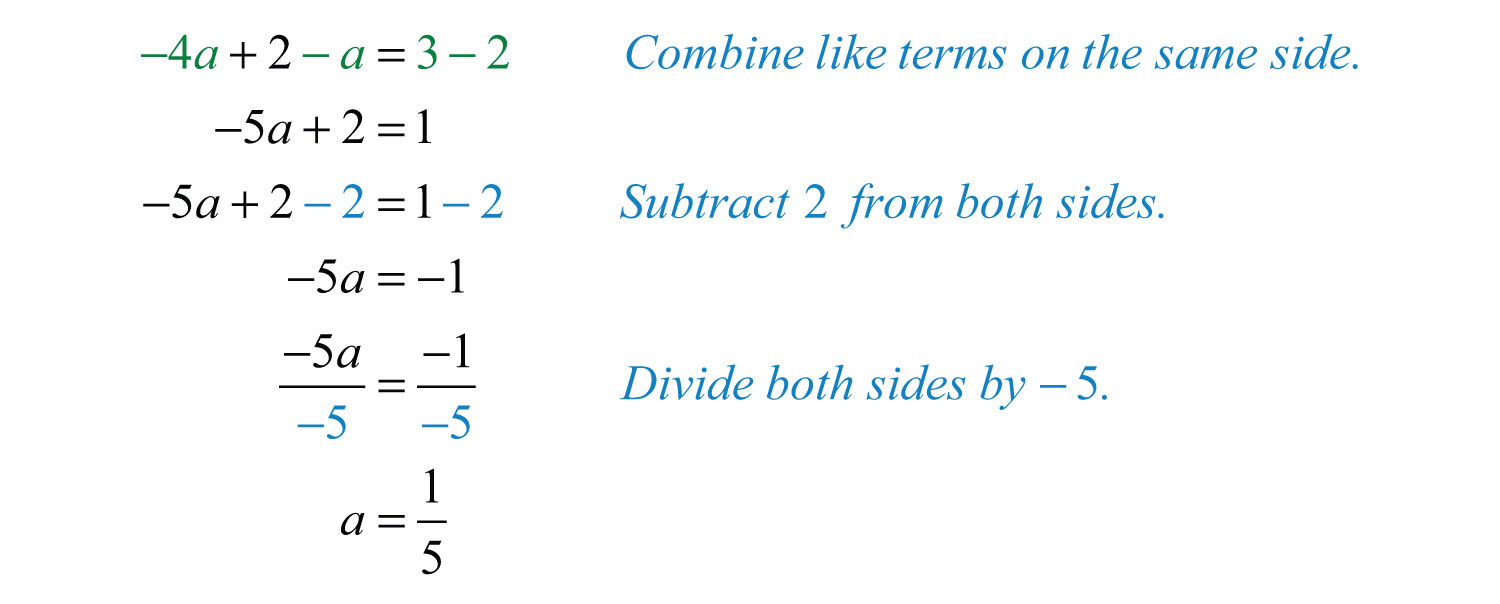Answer: The solution is $15$.

## Opposite-Side Like Terms

Given a linear equation in the form $ax+b=cx+d$, we begin by combining like terms on opposite sides of the equal sign. To combine opposite-side like termsLike terms of an equation on opposite sides of the equal sign., use the addition or subtraction property of equality to effectively “move terms” from one side to the other so that they can be combined.

Example 2: Solve: $−2y−3=5y+11$.

Solution: To “move” the term $5y$ to the left side, subtract it on both sides.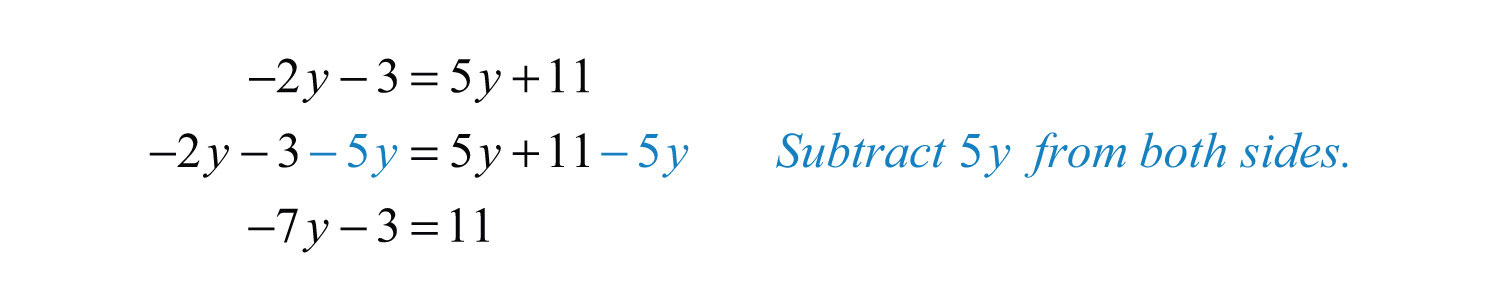From here, solve using the techniques developed previously.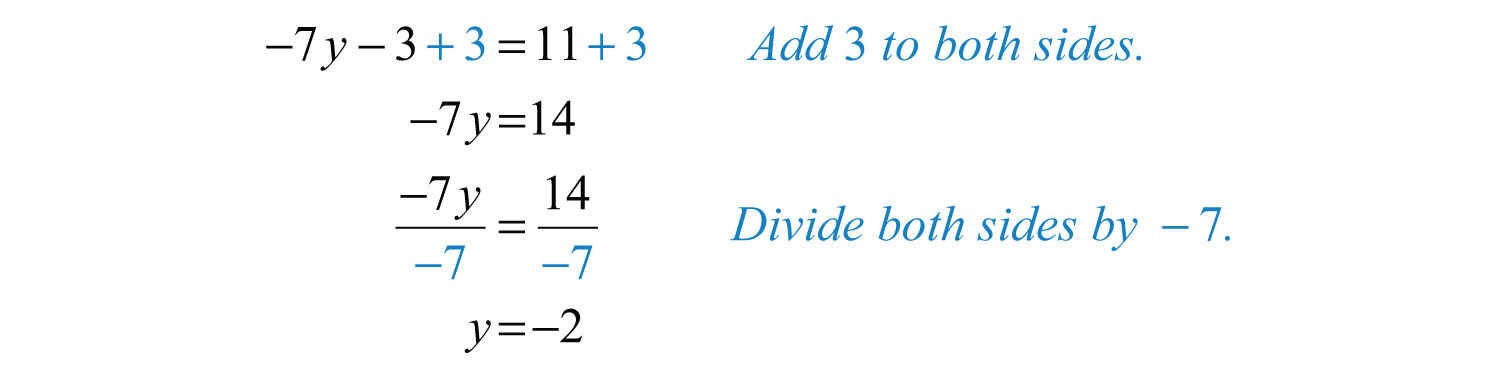Always check to see that the solution is correct by substituting the solution back into the original equation and simplifying to see if you obtain a true statement.## General Guidelines for Solving Linear Equations

When solving linear equations, the goal is to determine what value, if any, will produce a true statement when substituted in the original equation. Do this by isolating the variable using the following steps:

Step 1: Simplify both sides of the equation using the order of operations and combine all same-side like terms.

Step 2: Use the appropriate properties of equality to combine opposite-side like terms with the variable term on one side of the equation and the constant term on the other.

Step 3: Divide or multiply as needed to isolate the variable.

Step 4: Check to see if the answer solves the original equation.

Example 3: Solve: $−12(10y−2)+3=14$.

Solution: Simplify the linear expression on the left side before solving.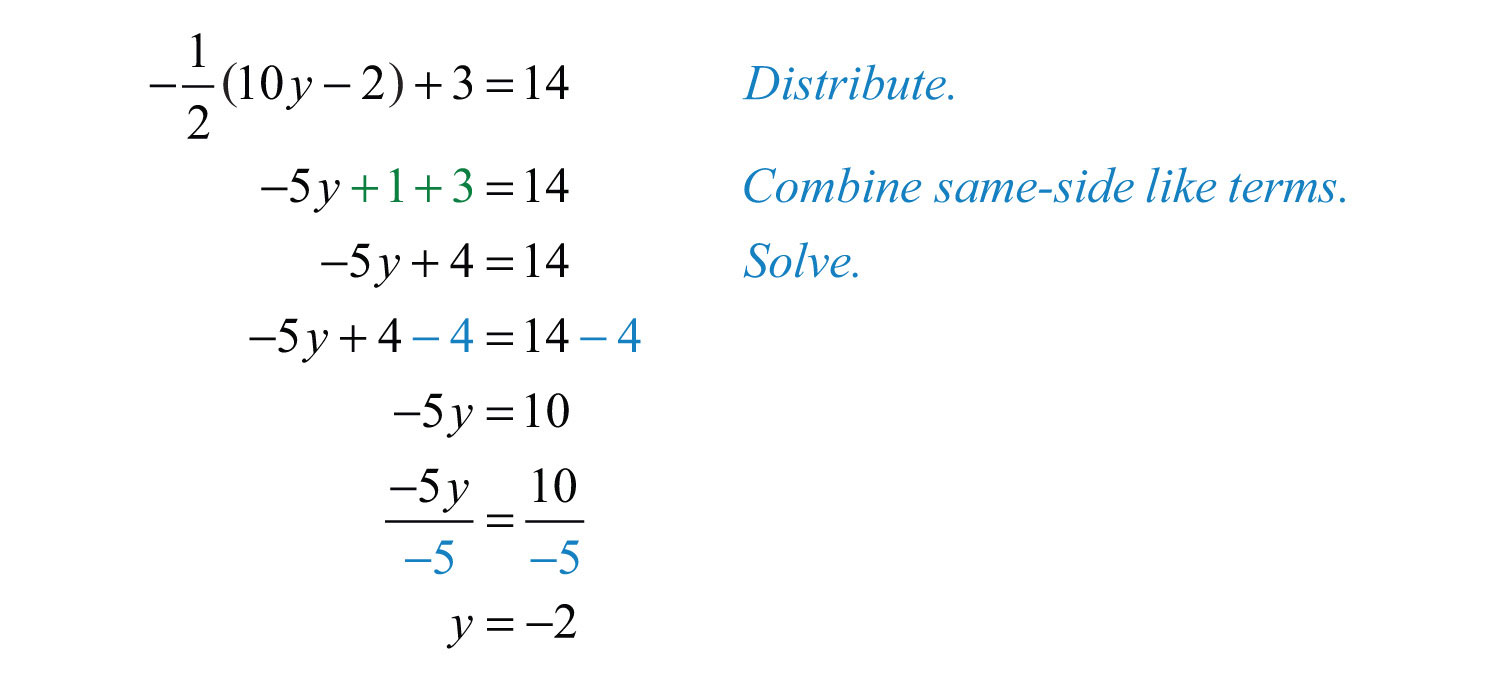To check,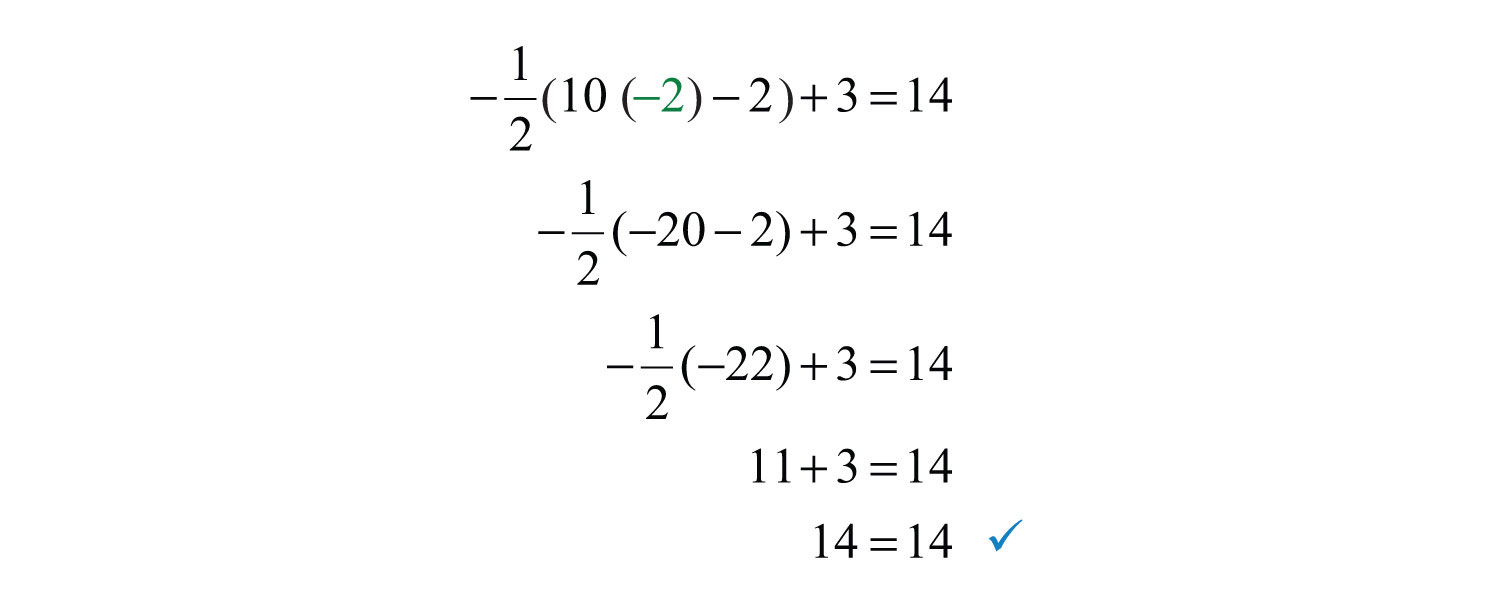Example 4: Solve: $5(3x+2)−2=−2(1−7x)$.

Solution: First, simplify the expressions on both sides of the equal sign.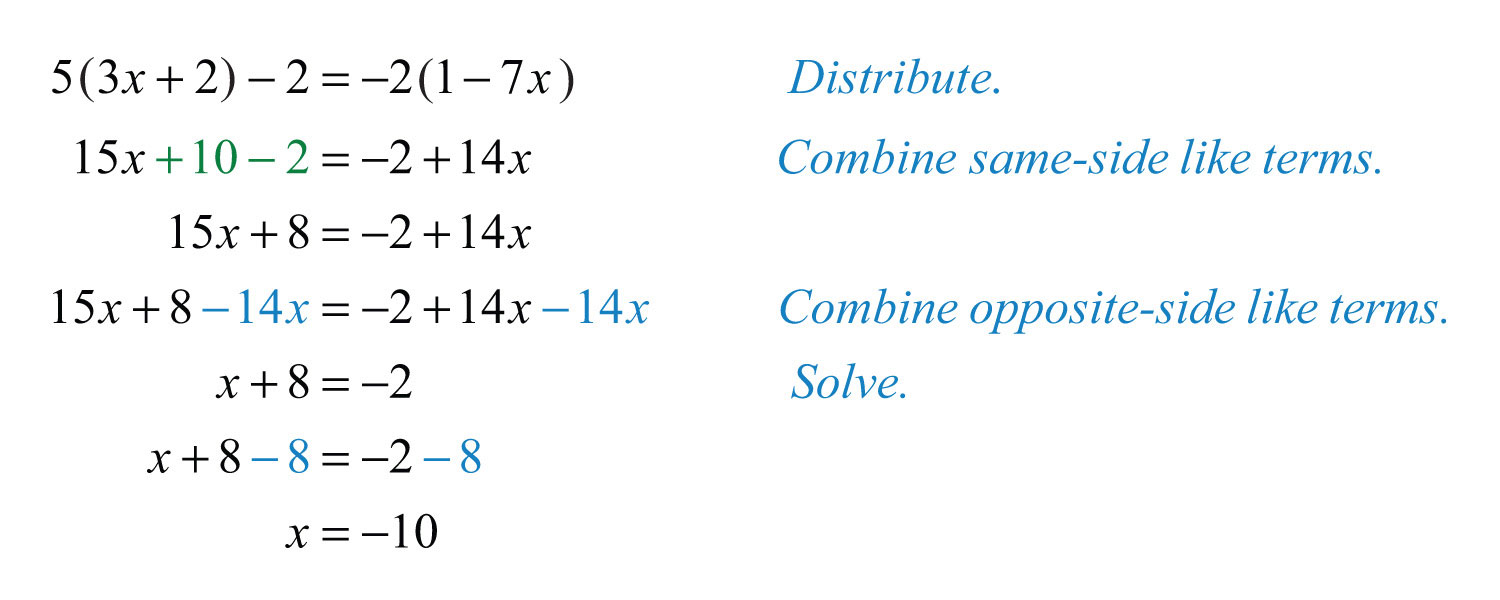Answer: The solution is −10. The check is left as an exercise.

Try this! Solve: $6−3(4x−1)=4x−7$.

Answer: $x=1$

### Video Solution

(click to see video)

## Conditional Equations, Identities, and Contradictions

There are three different types of equations. Up to this point, we have been solving conditional equationsEquations that are true for particular values.. These are equations that are true for particular values. An identityAn equation that is true for all possible values. is an equation that is true for all possible values of the variable. For example,has a solution set consisting of all real numbers, R. A contradictionAn equation that is never true and has no solution. is an equation that is never true and thus has no solutions. For example,has no solution. We use the empty set, $∅$, to indicate that there are no solutions.

If the end result of solving an equation is a true statement, like 0 = 0, then the equation is an identity and any real number is a solution. If solving results in a false statement, like 0 = 1, then the equation is a contradiction and there is no solution.

Example 5: Solve: $4(x+5)+6=2(2x+3)$.

Solution: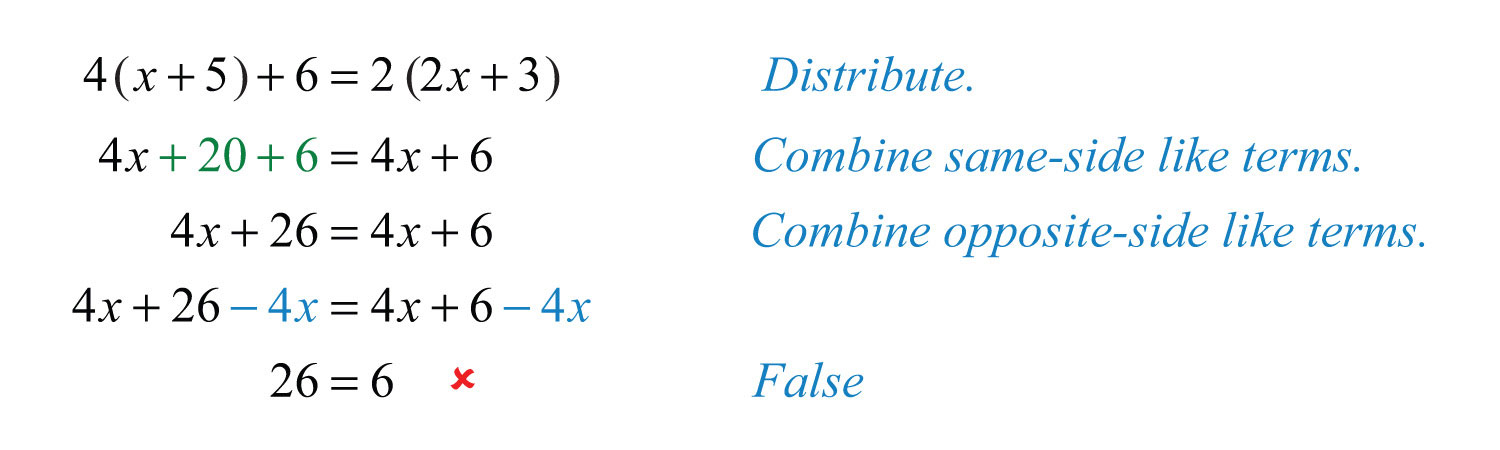Answer: $∅$. Solving leads to a false statement; therefore, the equation is a contradiction and there is no solution.

Example 6: Solve: $3(3y+5)+5=10(y+2)−y$.

Solution: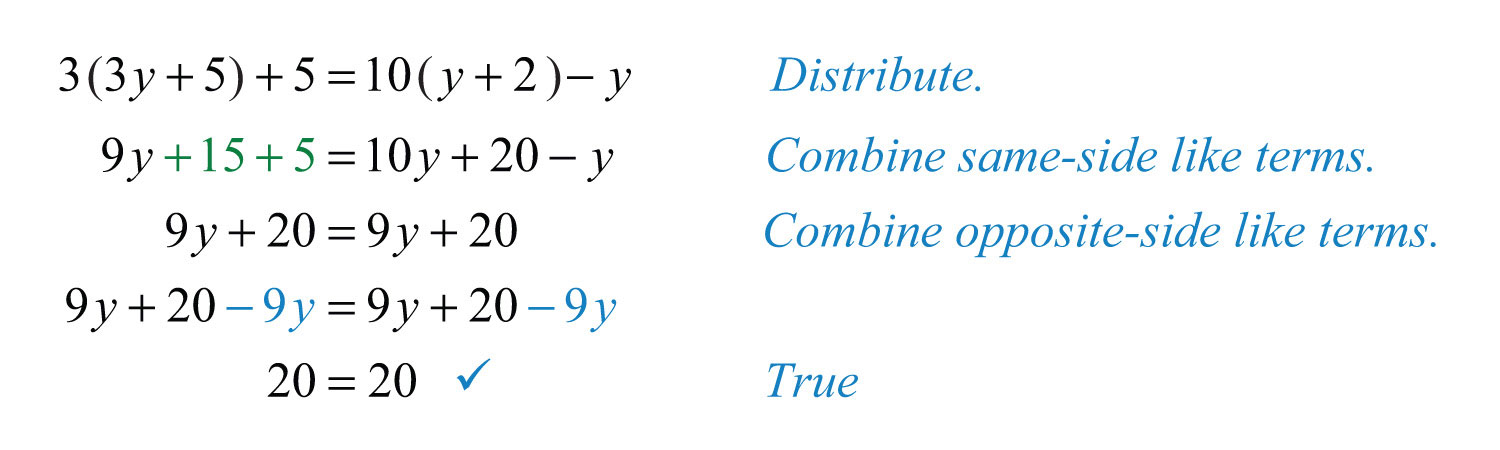Answer: R. Solving leads to a true statement; therefore, the equation is an identity and any real number is a solution.

If it is hard to believe that any real number is a solution to the equation in the previous example, then choose your favorite real number, and substitute it in the equation to see that it leads to a true statement. Choose $x=7$ and check:Try this! Solve: $−2(3x+1)−(x−3)=−7x+1$.

### Video Solution

(click to see video)

## Clearing Decimals and Fractions

The coefficients of linear equations may be any real number, even decimals and fractions. When decimals and fractions are used, it is possible to use the multiplication property of equality to clear the coefficients in a single step. If given decimal coefficients, then multiply by an appropriate power of 10 to clear the decimals. If given fractional coefficients, then multiply both sides of the equation by the least common multiple of the denominators (LCD).

Example 7: Solve: $2.3x+2.8=−1.2x+9.8$.

Solution: Notice that all decimal coefficients are expressed with digits in the tenths place; this suggests that we can clear the decimals by multiplying both sides by 10. Take care to distribute 10 to each term on both sides of the equation.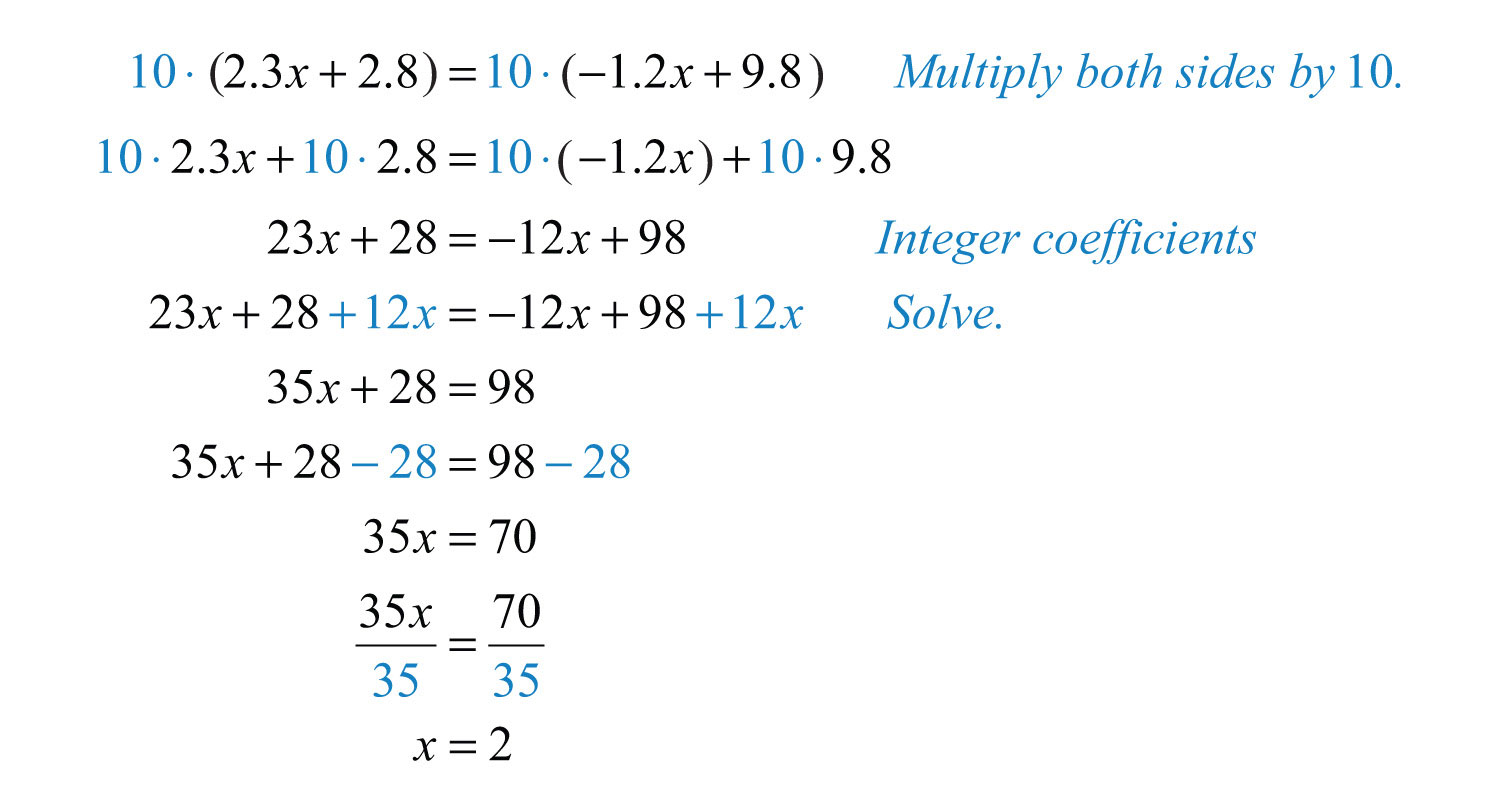Example 8: Solve: $13x+15=15x−1$.

Solution: Clear the fractions by multiplying both sides by the least common multiple of the given denominators. In this case, the .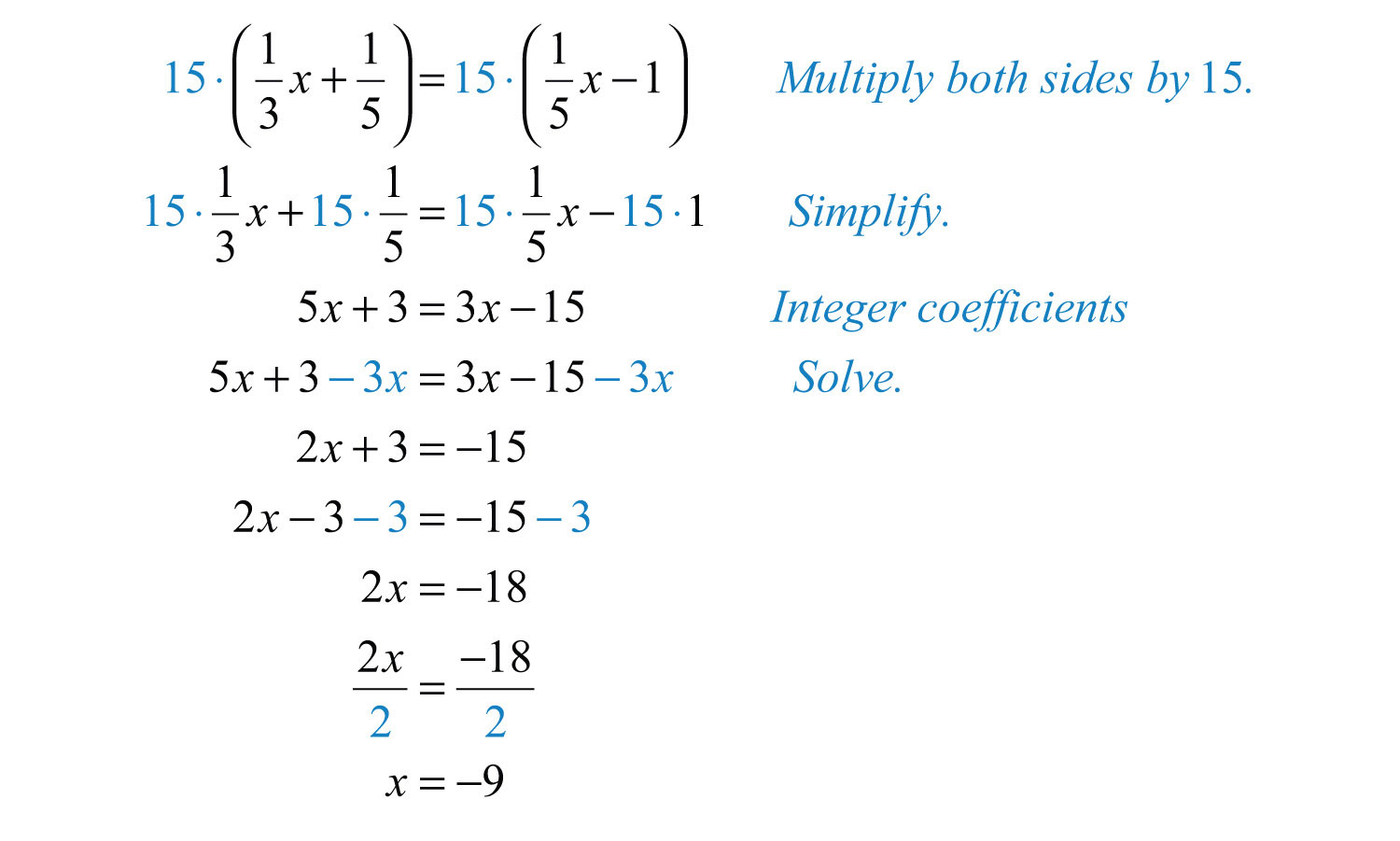It is important to know that these techniques only work for equations. Do not try to clear fractions when simplifying expressions. As a reminder,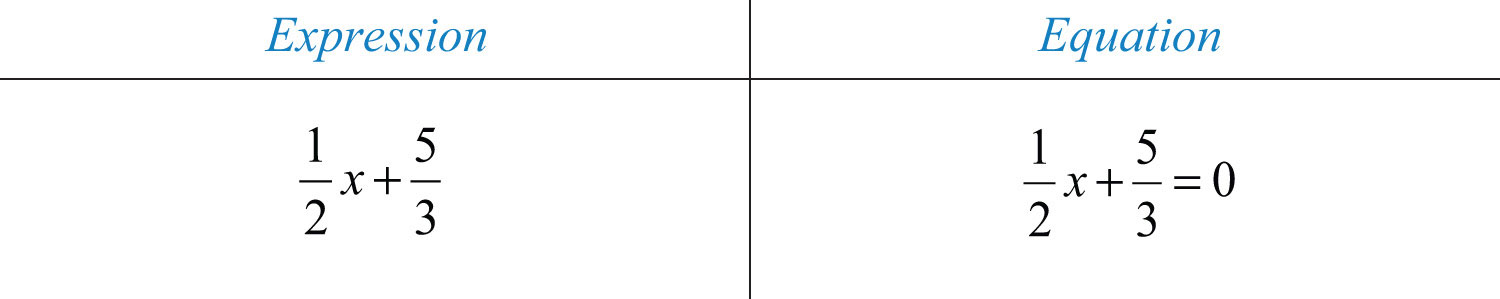Solve equations and simplify expressions. If you multiply an expression by 6, you will change the problem. However, if you multiply both sides of an equation by 6, you obtain an equivalent equation.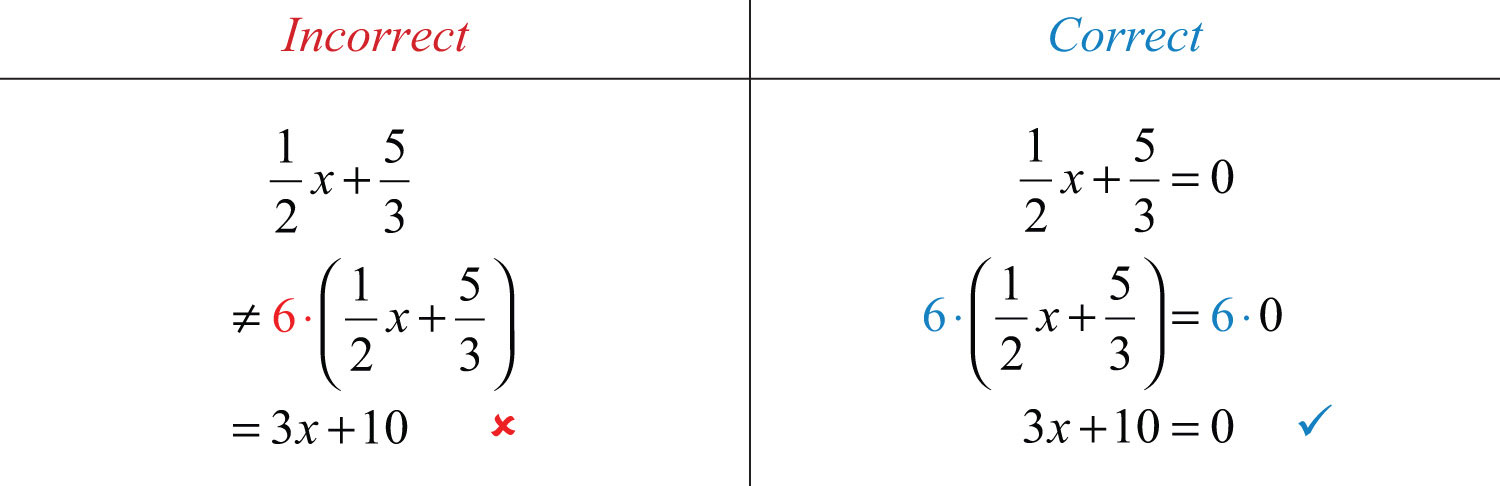## Literal Equations (Linear Formulas)

Algebra lets us solve whole classes of applications using literal equationsA formula that summarizes whole classes of problems., or formulas. Formulas often have more than one variable and describe, or model, a particular real-world problem. For example, the familiar formula $D=rt$ describes the distance traveled in terms of the average rate and time; given any two of these quantities, we can determine the third. Using algebra, we can solve the equation for any one of the variables and derive two more formulas.If we divide both sides by r, we obtain the formula $t=Dr$. Use this formula to find the time, given the distance and the rate.If we divide both sides by t, we obtain the formula $r=Dt$. Use this formula to find the rate, given the distance traveled and the time it takes to travel that distance. Using the techniques learned up to this point, we now have three equivalent formulas relating distance, average rate, and time:When given a literal equation, it is often necessary to solve for one of the variables in terms of the others. Use the properties of equality to isolate the indicated variable.

Example 9: Solve for a:   $P=2a+b$.

Solution: The goal is to isolate the variable a.Answer: $a=P−b2$

Example 10: Solve for y:   $z=x+y2$.

Solution: The goal is to isolate the variable y.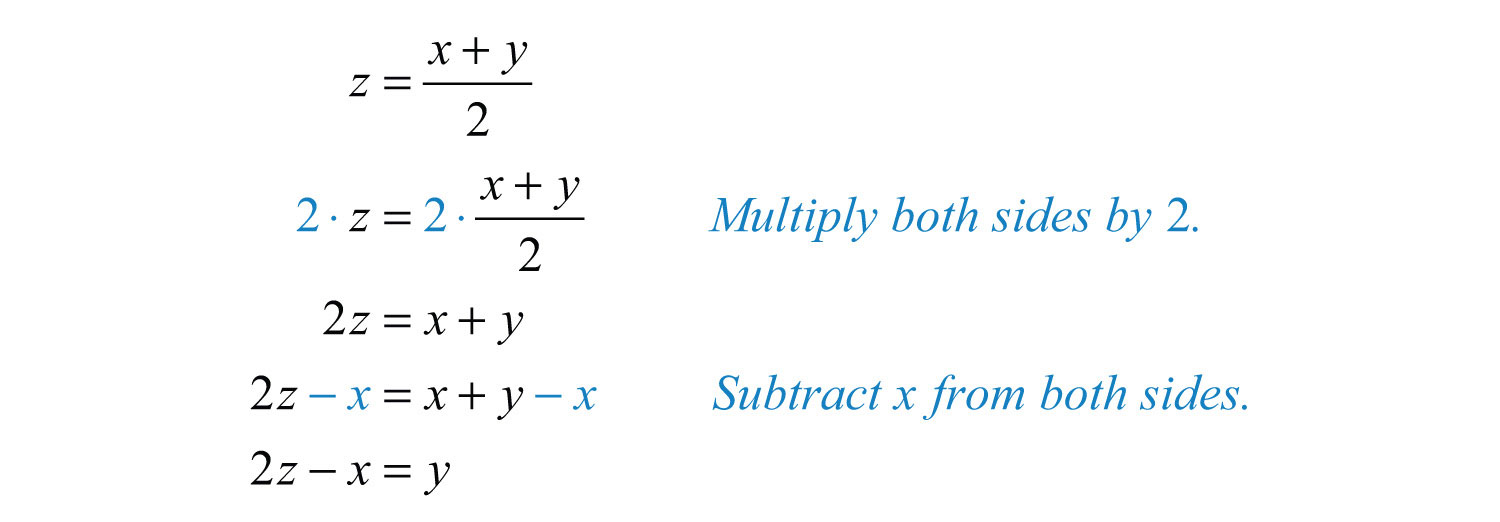Answer: $y=2z−x$

Try this! Solve for b:   $2a−3b=c$.

Answer: $b=2a−c3$

### Video Solution

(click to see video)

### Key Takeaways

• Solving general linear equations involves isolating the variable, with coefficient 1, on one side of the equal sign.
• The steps for solving linear equations are:

1. Simplify both sides of the equation and combine all same-side like terms.
2. Combine opposite-side like terms to obtain the variable term on one side of the equal sign and the constant term on the other.
3. Divide or multiply as needed to isolate the variable.
• Most linear equations that you will encounter are conditional and have one solution.
• If solving a linear equation leads to a true statement like 0 = 0, then the equation is an identity and the solution set consists of all real numbers, R.
• If solving a linear equation leads to a false statement like 0 = 5, then the equation is a contradiction and there is no solution, $∅$.
• Clear fractions by multiplying both sides of a linear equation by the least common multiple of all the denominators. Distribute and multiply all terms by the LCD to obtain an equivalent equation with integer coefficients.
• Given a formula, solve for any variable using the same techniques for solving linear equations. This works because variables are simply representations of real numbers.

### Topic Exercises

Part A: Checking for Solutions

Is the given value a solution to the linear equation?

1.

2.

3.

4.

5.

6.

Part B: Solving Linear Equations

Solve.

7. $4x−7=7x+5$

8. $−5x+3=−8x−9$

9. $3x−5=2x−17$

10. $−2y−52=3y+13$

11. $−4x+2=7x−20$

12. $4x−3=6x−15$

13. $9x−25=12x−25$

14. $12y+15=−6y+23$

15. $1.2x−0.7=3x+4.7$

16. $2.1x+6.1=−1.3x+4.4$

17. $2.02x+4.8=14.782−1.2x$

18. $−3.6x+5.5+8.2x=6.5+4.6x$

19. $12x−23=x+15$

20. $13x−12=−14x−13$

21. $−110y+25=15y+310$

22. $x−203=52x+56$

23. $23y+12=58y+3724$

24. $13+43x=107x+13−221x$

25. $89−1118x=76−12x$

26. $13−9x=49+12x$

27. $12x−5+9x=44$

28. $10−6x−13=12$

29. $−2+4x+9=7x+8−2x$

30. $20x−5+12x=6−x+7$

31. $3a+5−a=2a+7$

32. $−7b+3=2−5b+1−2b$

33. $7x−2+3x=4+2x−2$

34. $−3x+8−4x+2=10$

35. $6x+2−3x=−2x−13$

36. $3x−0.75+0.21x=1.24x+7.13$

37. $−x−2+4x=5+3x−7$

38. $−2y−5=8y−6−10y$

39. $110x−13=130−115x−715$

40. $58−43x+13=−39x−14+13x$

Part C: Solving Linear Equations Involving Parentheses

Solve.

41. $−5(2y−3)+2=12$

42. $3(5x+4)+5x=−8$

43. $4−2(x−5)=−2$

44. $10−5(3x+1)=5(x−4)$

45. $9−(x+7)=2(x−1)$

46. $−5(2x−1)+3=−12$

47. $3x−2(x+1)=x+5$

48. $5x−3(2x−1)=2(x−3)$

49. $−6(x−1)−3x=3(x+8)$

50. $−35(5x+10)=12(4x−12)$

51. $3.1(2x−3)+0.5=22.2$

52. $4.22−3.13(x−1)=5.2(2x+1)−11.38$

53. $6(x−2)−(7x−12)=14$

54. $−9(x−3)−3x=−3(4x+9)$

55. $3−2(x+4)=−3(4x−5)$

56. $12−2(2x+1)=4(x−1)$

57. $3(x+5)−2(2x+3)=7x+9$

58. $3(2x−1)−4(3x−2)=−5x+10$

59. $−3(2a−3)+2=3(a+7)$

60. $−2(5x−3)−1=5(−2x+1)$

61. $12(2x+1)−14(8x+2)=3(x−4)$

62. $−23(6x−3)−12=32(4x+1)$

63. $12(3x−1)+13(2x−5)=0$

64. $13(x−2)+15=19(3x+3)$

65. $−2(2x−7)−(x+3)=6(x−1)$

66. $10(3x+5)−5(4x+2)=2(5x+20)$

67. $2(x−3)−6(2x+1)=−5(2x−4)$

68. $5(x−2)−(4x−1)=−2(3−x)$

69. $6(3x−2)−(12x−1)+4=0$

70. $−3(4x−2)−(9x+3)−6x=0$

Part D: Literal Equations

Solve for the indicated variable.

71. Solve for w:   $A=l⋅w$.

72. Solve for a:   $F=ma$.

73. Solve for w:   $P=2l+2w$.

74. Solve for r:   $C=2πr$.

75. Solve for b:   $P=a+b+c$.

76. Solve for C:   $F=95C+32$.

77. Solve for h:   $A=12bh$.

78. Solve for t:   $I=Prt$.

79. Solve for y:   $ax+by=c$.

80. Solve for h:   $S=2πr2+2πrh$.

81. Solve for x:   $z=2x+y5$.

82. Solve for c:   $a=3b−2c3$.

83. Solve for b:   $y=mx+b$.

84. Solve for m:   $y=mx+b$.

85. Solve for y:   $3x−2y=6$.

86. Solve for y:   $−5x+2y=12$.

87. Solve for y:   $x3−y5=1$.

88. Solve for y:   $34x−15y=12$.

Translate the following sentences into linear equations and then solve.

89. The sum of 3x and 5 is equal to the sum of 2x and 7.

90. The sum of −5x and 6 is equal to the difference of 4x and 2.

91. The difference of 5x and 25 is equal to the difference of 3x and 51.

92. The sum of $12x$ and $34$ is equal to $23x$.

93. A number n divided by 5 is equal to the sum of twice the number and 3.

94. Negative ten times a number n is equal to the sum of three times the number and 13.

Part E: Discussion Board Topics

95. What is the origin of the word algebra?

96. What is regarded as the main business of algebra?

97. Why is solving equations such an important algebra topic?

98. Post some real-world linear formulas not presented in this section.

99. Research and discuss the contributions of Diophantus of Alexandria.

100. Create an identity or contradiction of your own and share on the discussion board. Provide a solution and explain how you found it.

1: Yes

3: No

5: Yes

7: −4

9: −12

11: 2

13: 0

15: −3

17: 3.1

19: −26/15

21: 1/3

23: 25

25: −5/2

27: 7/3

29: −1

31: $∅$

33: 1/2

35: −3

37: R

39: −3/5

41: 1/2

43: 8

45: 4/3

47: $∅$

49: −3/2

51: 5

53: −14

55: 2

57: 0

59: −10/9

61: 3

63: 1

65: 17/11

67: $∅$

69: 7/6

71: $w=Al$

73: $w=P−2l2$

75: $b=P−a−c$

77: $h=2Ab$

79: $y=−ax+cb$

81: $x=5z−y2$

83: $b=y−mx$

85: $y=3x−62$

87: $y=5x−153$

89: $3x+5=2x+7$; $x=2$

91: $5x−25=3x−51$; $x=−13$

93: $n5=2n+3$; $n=−53$